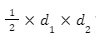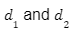×## Introduction

Rhombus and Parallelogram both belong to the quadrilateral family as they have four sides each. But there are major differences between these two quadrilaterals in terms of their angles and their sides. The rhombus is an equilateral figure whereas the parallelogram is neither equilateral nor equiangular. In a rhombus, all four edges are identical in length and pairwise opposite edges are parallel but in a parallelogram opposite edges are identical lengthwise. Talking about the diagonal property, in rhombus diagonals intersect at right angles and bisect one another but in a parallelogram diagonals only bisect one another. Hence in this article, we discuss all the differences between rhombus and parallelogram.

## A rhombus is a quadrilateral whose opposite edge pairs are parallel and whose opposite angles are equal to one another. All of its edges are the same length.The primary difference between a square and a rhombus is that a square has all of its angles equivalent to 90 degrees while a rhombus does not. Additionally, not all squares are rhombus, but all rhombus are squares.

The following are some of a rhombus' significant characteristics:

• A rhombus's diagonals are perpendicular to one another and bisect one another. (forms right angle). Scalene shapes are formed by them.

• The rhombus's interior angles are divided by a diagonal, and its opposite angles are identical.

• The lengths of adjacent edges are identical.

Parallelogram

A particular kind of quadrilateral is a parallelogram. A parallelogram is a quadrilateral with pairs of opposite edges that are equal and parallel. A parallelogram with equal-sized edges is known as a rhombus.Several important features of a parallelogram include:

• The opposing edges are parallel to one another and have identical lengths.

• The opposing lines are split by diagonals.

• Diagonals cut each other in half.

• The parallelogram is divided into two identical triangles  by each diagonal.

## Differences Between Rhombus and Parallelogram

Rhombus and parallelogram are different in the following sense

 Rhombus Parallelogram In a rhombus all sides are equal in length. The pair of sides that are opposite to each other are equal in length. The diagonals bisect each other perpendicularly Diagonals in a parallelogram are solely their own bisectors. Scalene triangles are those that have a common side that is a diagonal. Triangles formed with diagonals as their common sides are congruent to one another. The lengths of a rhombus' diagonals are used to calculate its area. The lengths of base and height  are used to evaluate the area of parallelogram Area of the rhombus is given bywhere,are length of diagonals. The area of the parallelogram is given by b 🇽 h where is b base and h is the height of the given parallelogram If the side length of rhombus is a then its perimeter is 4a. Let a and b are two adjacent sides of the parallelogram, then its perimeter is given by 2(a+b).If AB=BC=CD=AD also AC and BD are diagonals then the figure is a rhombus.If PQ = SR and PS= QR followed by both opposite sides are parallel then PQRS is a parallelogram.

How to Identify Whether a Quadrilateral is Rhombus or a Parallelogram?

A quadrilateral will be a rhombus if all of its edges have the same length and all of its opposite angles have the same size. A parallelogram is formed when the opposite edges and angles of a quadrilateral have the same length and measure, respectively. Every parallelogram can be thought of as a rhombus, although not all parallelograms are rhombuses. A parallelogram will turn into a rhombus if all of its sides are equal. The characteristics of a rhombus and a parallelogram are listed below to help you tell them apart.

• In a parallelogram, the opposing edges are equal in length, the opposing edges are parallel, the opposing angles are equal in size, and the inner angles on the same side are supplementary. The parallelogram is divided into two congruent triangles by each of its diagonals. A parallelogram's diagonals are bisectors of one another.
• In a rhombus, all edges are the same length, all opposed angles are the same size, and opposite sides are parallel to one another. Diagonals are each other's perpendicular bisectors. Any two neighboring angles added together make 180 degrees.

## Summary

A figure with 4 equal sides with mutually perpendicular diagonals is a rhombus. Parallelogram is a 4-sided figure with lengths of its pairs of opposite sides the same. Rhombus and parallelogram belong to the family of quadrilaterals and both these figures have few differences. Every rhombus is a parallelogram but every parallelogram cannot be a rhombus

What makes a rhombus a parallelogram?

All four sides of a rhombus are congruent, which means that opposing sides are identical, which is one of the characteristics of a parallelogram. The other two sides can be done in the same way, and we already know that the opposing sides are parallel.

2. Which geometric figure can be regarded as both a parallelogram  and a rhombus?

Ans: A square is a geometric shape that can be classified as a parallelogram and a rhombus because pairs of opposing edges can be thought of as equal and parallel.3. Are the diagonals of a rhombus equal?

The Rhombus is then a quadrilateral whose diagonals are not equivalent and bisect one another.

#### Recent Posts